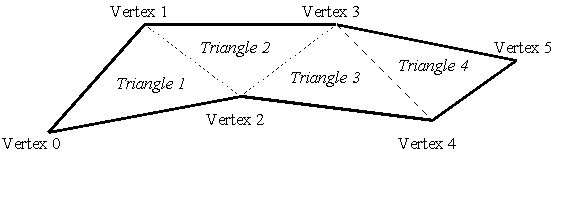Draw Polytriangle

Purpose

The Draw Polytriangle function draws a connected chain of triangles.

Syntax

 Opcode format Opcode [ASCII] (Hex) Operand Format Comments Extended ASCII TBD [][,]+ Absolute coordinates. Single-byte, binary operand [t] (74) [][]+ Relative coordinates. [Ctrl-T] (14) [][]+ Relative coordinates. Extended binrary TBD [][]]+ Gouraud, relative coordinates. TBD [][]+ Texture mapped, relative coordinates. TBD [][]+ Gouraud and texture mapped, relative coordinates.

Count The number of points in the polytriangle. When using the binary operand form, a value of zero for count indicates that an extended count will follow. In the ASCII operand case, count may be any value larger than zero.

Ecount When count is zero, a 2-byte extended count follows, allowing for polytriangles with vertices from 256 through 65,791 which are encoded as an integer (0 to 65,535).

Xi,Yi The ith point (in logical coordinates) of the polytriangle.

Iid Selects an image previously defined with Draw Image for use with texture mapping.

Ri, Gi, Bi, Ai The color definition at the ith vertex in the polytriangle.

Ui, Vi, Wi The texture coordinate at the ith vertex in the polytriangle. The coordinate selects colors from the image selected by the Iid parameter.

Details

Topologically, a polytriangle is a strip of connected triangles, where each successive triangle in the chain is defined by a single vertex and two vertices from the previous triangle in the strip, as shown in figure 1.Figure 1. Example polytriangle with six points

A polytriangle is similar to a polygon, but the vertices are specified in a special order.

When texture mapping, the Ui and Vi parameters correspond to the horizontal and vertical directions of texture space, and Wi corresponds to the perspective homogeneous coordinate (used when a 2D projection is made from a 3D object). The 32-bit value for Ui and Vi are interpreted as a signed fixed-point texture parameter: the low 16-bits correspond to the normalized fractional portion of the texture image, and the high 16-bits correspond to the integer portion.

Notes

If a polytriangle with more than 65,791 triangles is desired, it must be drawn by multiple Draw Polytriangle opcodes.

Implementation in WHIP! Versions

 Toolkit Function TBD Partial Full Revised in Draw Polytriangle - 0.5x, 1.03 - 2.0